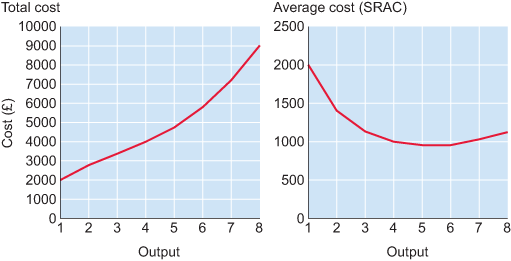Economics and the 2008 crisis: a Keynesian view

Start this free course now. Just create an account and sign in. Enrol and complete the course for a free statement of participation or digital badge if available.

Short run average cost (SRAC) curves (2)

It’s often easier to represent cost curves graphically. Here the two columns of data in Table 4 are shown in separate graphs. The table is repeated below.Figure 34 Total cost and average cost curves
Quantity of output (units)Total cost (£)Average cost (£/unit)
120002000
228001400
334001133
440001000
54750950
65800967
772001029
890001125

Notice that total cost increases with output, albeit at varying rates. The SRAC captures how average cost (or unit cost) changes with output. When output is low, initially average cost falls, but as output increases, average cost starts to rise. Next let’s examine the components of average cost – fixed and variable cost.